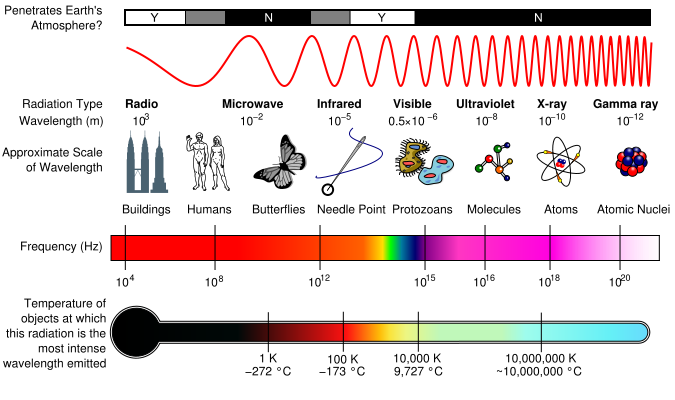# Wave characteristics

## 4.4.1 Describe a wave pulse and a continuous progressive (travelling) wave.

A wave is a disturbance that propagates through some material medium or space.

Waves have the following characteristics:

• They all transfer energy
• They do this without a net motion
• They all involve oscillations that are SHM

A wave pulse involves just one oscillation

A continuous wave involves a succession of individual oscillations.

## 4.4.2 State that progressive (travelling) waves transfer energy.

Waves transfer energy without the net motion of the medium through which the wave travels.

## 4.4.3 Describe and give examples of transverse and of longitudinal waves.

Transverse waves are waves in which the oscillations of the medium are at right angles to the direction of the energy transfer, e.g. light waves.

Longitudinal waves are waves in which the oscillations are parallel to the direction of energy transfer, e.g. sound waves.

## 4.4.4 Describe waves in two dimensions, including the concepts of wavefronts and of rays.

Take for example a water wave. The wavefronts are the parts of the wave that are moving together. Rays represent the direction of the energy transfer.

## 4.4.5 Describe the terms crest, trough, compression and rarefaction.

Crest - The top, maximum height of the transverse wave

Trough - The bottom, the lowest point, of the transverse wave.

Compression - A point on the longitudinal wave that are "bunched together" (high pressure)

Rarefraction - A point on the longitudinal wave that are "far apart" (low pressure)

## 4.4.6 Define the terms displacement, amplitude, frequency, period, wavelength, wave speed and intensity.

Displacement: The amount by which a particle is moved from equilibrium position.

Amplitude: The maximum displacement of a particle from its equilibrium position.

Period: The time that it takes a particle to make one complete oscillation, or the time that it takes for the wave to travel a complete wavelength.

Wavelength: The shortest distance along a wave between two points that are "in phase" with one another. e.g the distance from one crest to another crest on a water wave.

Wave speed: The speed at which the wave fronts pass a stationary object.

Intensity: The intensity of the wave is the power per unit area that is received by a stationary observer.  intensity ∝ amplitude2.

## 4.4.8 Derive and apply the relationship between wave speed, wavelength and frequency.## 4.4.9 State that all electromagnetic waves travel with the same speed in free space, and recall the orders of magnitude of the wavelengths of the principal radiations in the electromagnetic spectrum.Figure 4.4.1 - The electromagnetic spectrum